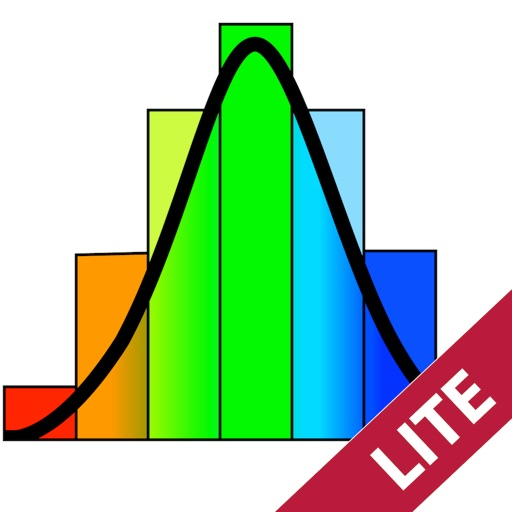## MATH GRAPHING 2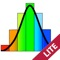# Math Graphing Lite - Dynamic Scientific Calculator

by KYBERVISION JAPAN LLC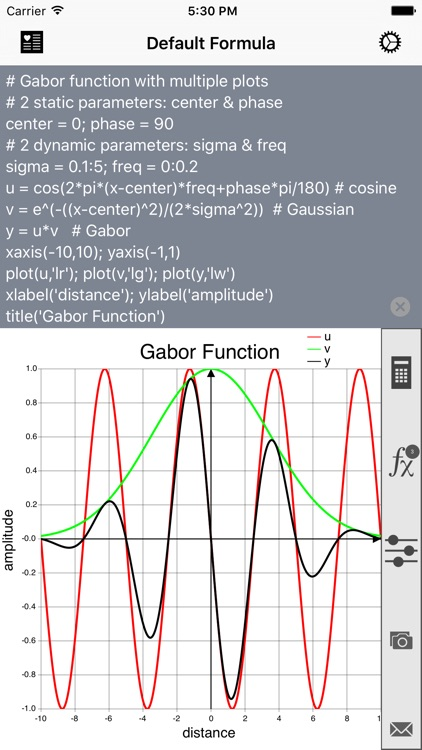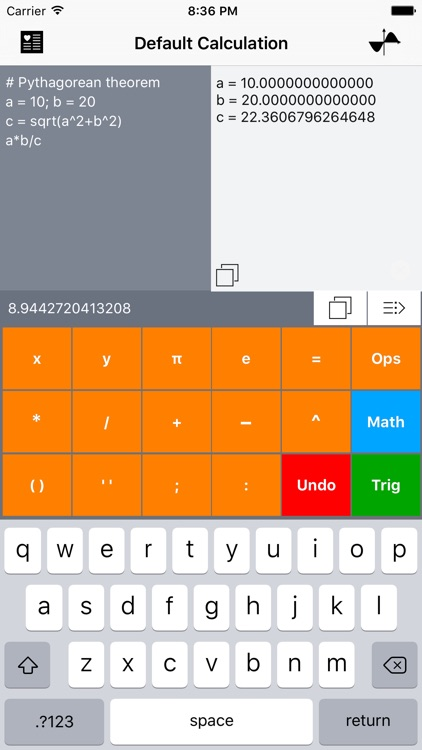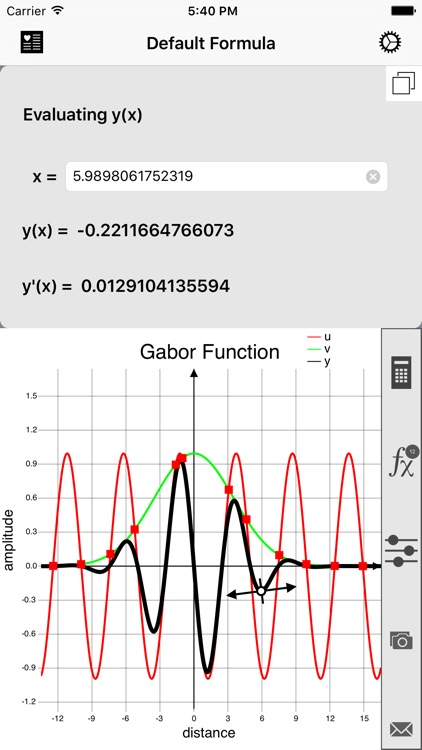MATH GRAPHING 2.0 is a unique graphing app to plot simultaneously multiple mathematical expressions of arbitrary complexity using static and dynamic variables (including a time variable and random generation functions). It also includes a standard calculator mode that allows simultaneous evaluations of any number of intermediary variables.### App Details

Version
2.0.1
Rating
(3)
Size
6Mb
Genre
Education Utilities
Last updated
December 20, 2016
Release date
September 28, 2009

### Math Graphing Lite is FREE but there are more add-ons

• \$4.99

PowerPack

• \$1.99

• \$2.99

Calculator Pack

### App Screenshots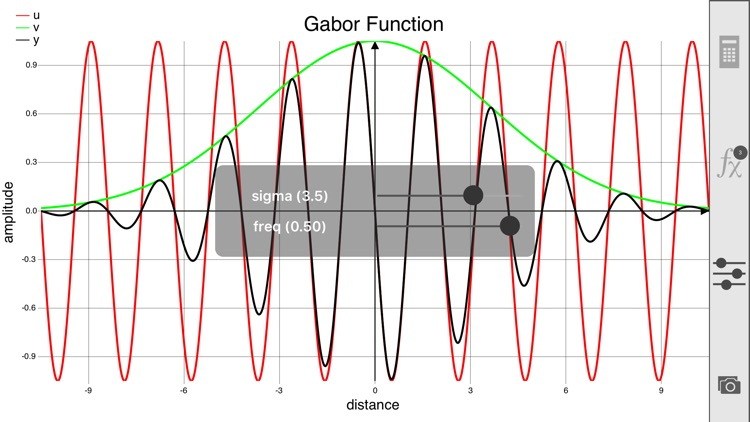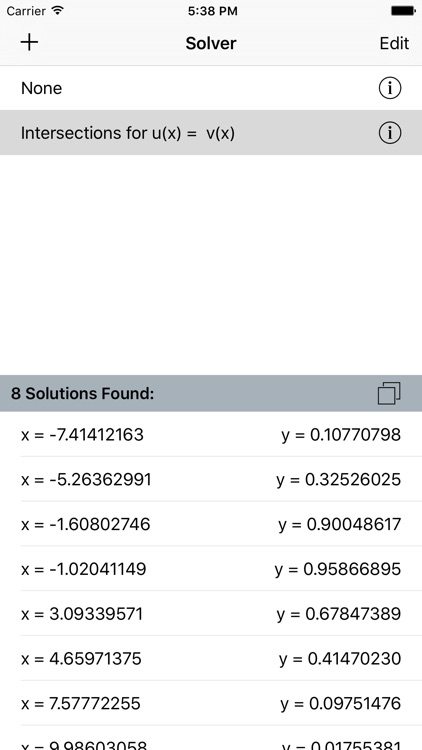### App Store Description

MATH GRAPHING 2.0 is a unique graphing app to plot simultaneously multiple mathematical expressions of arbitrary complexity using static and dynamic variables (including a time variable and random generation functions). It also includes a standard calculator mode that allows simultaneous evaluations of any number of intermediary variables.

"Math Graphing" can help students improve their math skills by developing some visual intuition of mathematical expressions or advanced users who need some scientific capabilities only available with expensive desktop software.

MATH GRAPHING provides the following functionalities:

- Multiple expressions with quasi-unlimited number of variables can be combined to produce simple or complex formulas.
- Interactive sliders can be created to visually investigate the role of important parameters on the graphical representation of the formulas.
- Trace mode to display coordinates and derivative of marker on selected curve.
- Landscape mode for graphing.
- Solver tool to solve y = f(x) where x or y are unknown, local/global minima/maxima/extrema or intersections g(x) = f(x).
- Formula graphs can be saved to the device's Photos Album and the formulas can be exported through email with embedded graphs.
- Add any formula or calculation to your list of favorites (in-app purchase).
- Several formulas can be plotted simultaneously in different colors and styles.
- Customizable graph appearance: axes labels and ranges, title, curve color and style, ticks number, grid, legends, etc.
- A single-precision calculator supporting multiple variables.

Example of mathematical expression:

# Gabor function with multiple plots
# 2 static parameters: center & phase
center = 0; phase = 90
# 2 dynamic parameters: sigma & freq
sigma = 1:50; freq = 0:0.2
u = cos(2*pi*(x-50)*freq+phase*pi/180)
v = e^(-((x-center)^2)/(2*sigma^2))
y = u*v
plot(u,'lr'); plot(v,'lg'); plot(y,'lw')
xlabel('distance'); ylabel('amplitude')
title('Gabor Function')